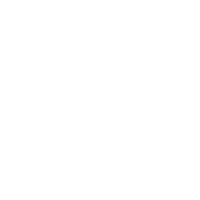# NUMBERS RIDDLES WITH ANSWERS TO SOLVE - PUZZLES & BRAIN TEASERS

#### Popular Searches

Terms · Privacy · Contact

## Numbers Riddles To Solve## Solving Numbers Riddles

Here we've provide a compiled a list of the best numbers puzzles and riddles to solve we could find.

Our team works hard to help you piece fun ideas together to develop riddles based on different topics. Whether it's a class activity for school, event, scavenger hunt, puzzle assignment, your personal project or just fun in general our database serve as a tool to help you get started.

Here's a list of related tags to browse:

The results compiled are acquired by taking your search "numbers" and breaking it down to search through our database for relevant content.

Browse the list below:

## The Fourth Column Riddle

Hint:
The correct choice will be the option 'd'.

In each succeeding row, the previous column is reversed and the lowest digits are omitted.
Did you answer this riddle correctly?
YES  NO
Solved: 58%

## Find Those Missing Numbers Riddle

Hint:
The number missing on the left is 8 and the one on right is 7.

Explanation:
The pattern:
The number in the center of triangle is the square root of the sum of the numbers sitting outside on the corners.

Thus,
10 + 22 + 4 = 36
Square Root of 36 = 6

In the same manner:

10 + 42 + 12 = 64
Square Root of 64 = 8

12 + 22 + 15 = 49
Square Root of 49 = 7
Did you answer this riddle correctly?
YES  NO
Solved: 29%

## 7 Guys 6 Rooms Riddle

Hint:
He never did anything with the 7th guy.
Did you answer this riddle correctly?
YES  NO
Solved: 54%

## On Display In December

Hint:
Did you answer this riddle correctly?
YES  NO
Solved: 54%

## The Countdown Is On

Hint:
Did you answer this riddle correctly?
YES  NO

## The Blue And Red Dice Riddle

Hint:
Each die has 6 faces. When two dice are thrown, there are 36 equally possible results. For chances to be even, there must be 18 ways of getting the same color on top. Let X be the number of red faces on the second die. We have: 18 = 5X + 1(6 - X)

X = 3

The second die must have 3 red faces and 3 blue faces.
Did you answer this riddle correctly?
YES  NO
Hint: Their dad is a very smart person.
Believe it or not, both Mike and James have a 1/2 chance of winning.

James wins if:
-he calls both coin flips right = 1/2 x 1/2 = 1/4
OR
-he does not call both coin flips right, Mike does not call the die roll correctly, and he guesses the number on the spinner right = 3/4 x 5/6 x 2/5 = 30/120 = 1/4

1/4 + 1/4 = 1/2

Mike wins if:
-James does not call both coin flips right and he calls the die roll correctly = 3/4 x 1/6 = 3/24 = 1/8
OR
-James does not call both coin flips right, he does not call the die roll correctly, and Mike does not guess the number on the spinner right = 3/4 x 5/6 x 3/5 = 45/120 = 3/8

1/8 + 3/8 = 1/2

Of course, dad could have just flipped a coin
Did you answer this riddle correctly?
YES  NO

## 100 Offices Riddle

Hint:
Did you say three? The correct answer is twenty (29, 39, and so on).
Did you answer this riddle correctly?
YES  NO
Solved: 63%
Hint:
He has only gotten one fortune cookie.
Did you answer this riddle correctly?
YES  NO
Solved: 74%

## The Ball Pyramid Riddle

Hint:
30

Explanation:
It's very difficult to count an actual number of balls but it can be counted mathematically as illustrated below.

Balls in lowest level most level, say level 1 : 4 * 4 = 16
Level2 => 3 * 3 = 9
Level3 => 2 * 2 = 4
Leve4 => 1

Summing up 16+9+4+1 = 30.
Did you answer this riddle correctly?
YES  NO
Solved: 69%